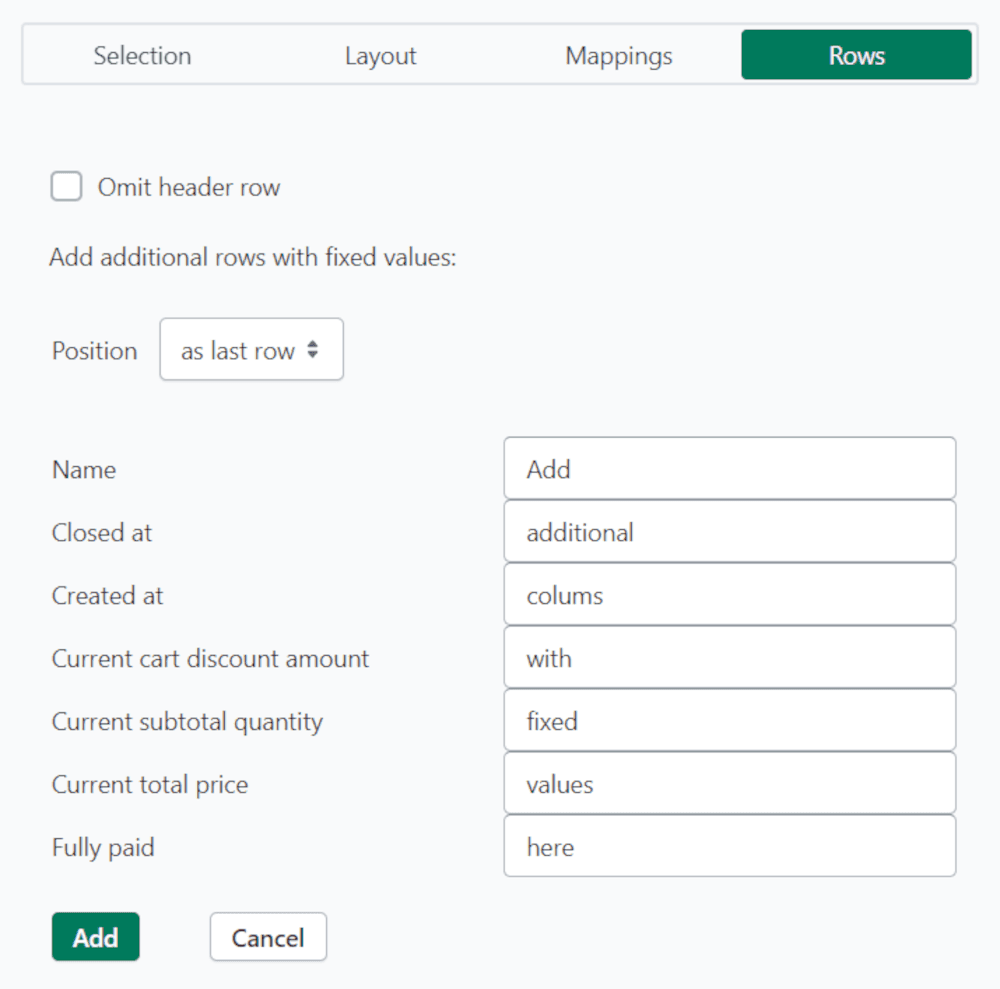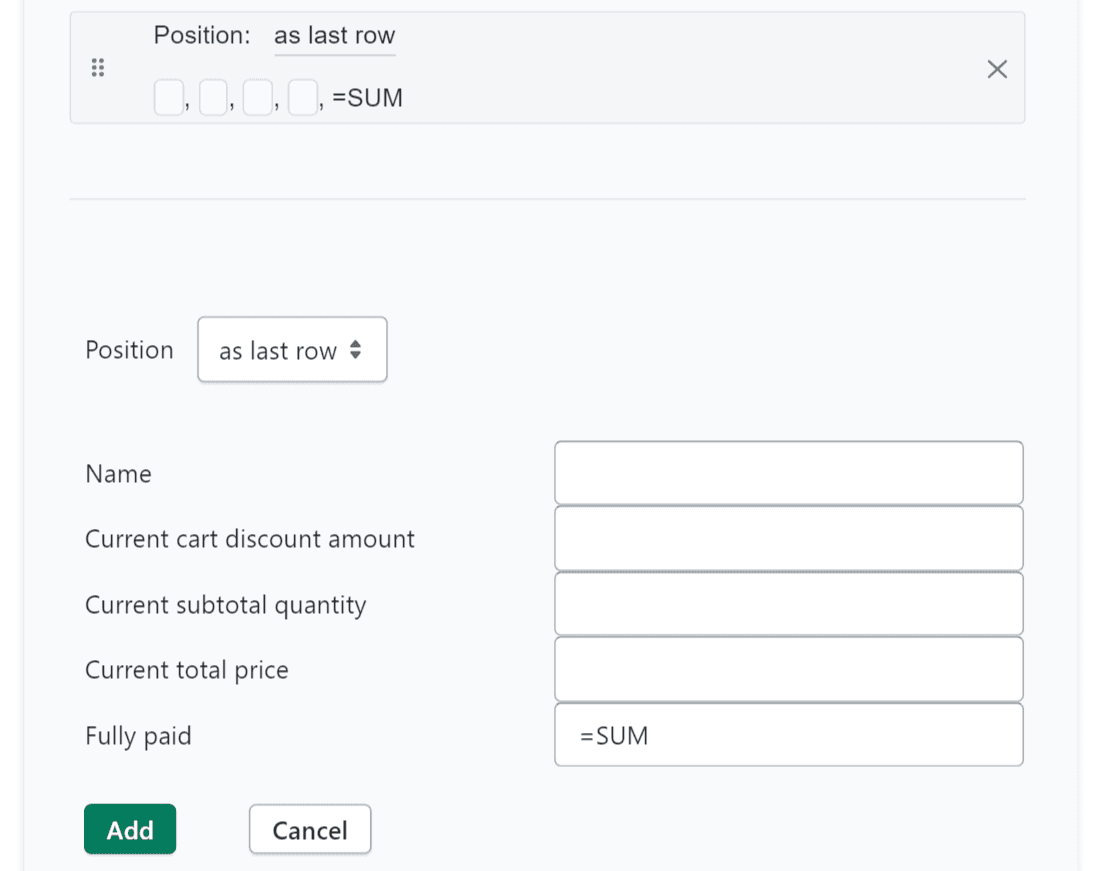Report Editor

Rows

Rows

How to add and customize rows

How to add and customize rows

Written By: Marvin Hödlmoser

Last Updated on August 23, 2023

### Rows

• "Omit header row": No header row will be in the output file (Excel, CSV)

• as first row

• as last row

• before each new item

• after each new itemIf you use the position "before each new item" or "after each new item" you can access the values from other columns in the row, do calculations or do advanced search and replace. Please take a look at:

Set the value of a column using values of other columns and using math expressions

#### Use Excel formulas to calculate sum, average, ...

This will work only for rows "as last row".

You can use any Excel formula that will calculate the content from the column above (like SUM or AVERAGE do). As in Excel start your input with a "=" like: "=SUM", "=AVERAGE" or "=COUNT"

You can add as many "as last rows" as you want - like in this example: